This tour explores the use of gradient descent method for unconstrained and constrained optimization of a smooth function

Important: Please read the installation page for details about how to install the toolboxes. $\newcommand{\dotp}{\langle #1, #2 \rangle}$ $\newcommand{\pd}{ \frac{ \partial #1}{\partial #2} }$ $\newcommand{\umin}{\underset{#1}{\min}\;}$ $\newcommand{\qandq}{\quad\text{and}\quad}$ $\newcommand{\qwhereq}{\quad\text{where}\quad}$ $\newcommand{\qifq}{ \quad \text{if} \quad }$ $\newcommand{\ZZ}{\mathbb{Z}}$ $\newcommand{\RR}{\mathbb{R}}$ $\newcommand{\pa}{\left(#1\right)}$ $\newcommand{\si}{\sigma}$ $\newcommand{\Nn}{\mathcal{N}}$ $\newcommand{\Hh}{\mathcal{H}}$ $\newcommand{\Bb}{\mathcal{B}}$ $\newcommand{\EE}{\mathbb{E}}$ $\newcommand{\norm}{\|#1\|}$ $\newcommand{\abs}{\left|#1\right|}$ $\newcommand{\choice}{ \left\{ \begin{array}{l} #1 \end{array} \right. }$ $\newcommand{\al}{\alpha}$ $\newcommand{\la}{\lambda}$ $\newcommand{\ga}{\gamma}$ $\newcommand{\Ga}{\Gamma}$ $\newcommand{\La}{\Lambda}$ $\newcommand{\si}{\sigma}$ $\newcommand{\Si}{\Sigma}$ $\newcommand{\be}{\beta}$ $\newcommand{\de}{\delta}$ $\newcommand{\De}{\Delta}$ $\newcommand{\phi}{\varphi}$ $\newcommand{\th}{\theta}$ $\newcommand{\om}{\omega}$ $\newcommand{\Om}{\Omega}$

In :
options(warn=-1) # turns off warnings, to turn on: "options(warn=0)"

library(imager)
library(png)
library(pracma)

# Importing the libraries
for (f in list.files(path="nt_toolbox/toolbox_general/", pattern="*.R")) {
source(paste("nt_toolbox/toolbox_general/", f, sep=""))
}
for (f in list.files(path="nt_toolbox/toolbox_signal/", pattern="*.R")) {
source(paste("nt_toolbox/toolbox_signal/", f, sep=""))
}

Loading required package: plyr

Attaching package: 'imager'

The following object is masked from 'package:magrittr':

The following object is masked from 'package:plyr':

liply

The following objects are masked from 'package:stats':

convolve, spectrum

The following object is masked from 'package:graphics':

frame

The following object is masked from 'package:base':

save.image

Attaching package: 'pracma'

The following objects are masked from 'package:magrittr':

and, mod, or

Attaching package: 'tuneR'

The following objects are masked from 'package:imager':

channel, play

Attaching package: 'akima'

The following object is masked from 'package:imager':

interp



## Gradient Descent for Unconstrained Problems¶

We consider the problem of finding a minimum of a function $f$, hence solving $$\umin{x \in \RR^d} f(x)$$ where $f : \RR^d \rightarrow \RR$ is a smooth function.

Note that the minimum is not necessarily unique. In the general case, $f$ might exhibit local minima, in which case the proposed algorithms is not expected to find a global minimizer of the problem. In this tour, we restrict our attention to convex function, so that the methods will converge to a global minimizer.

The simplest method is the gradient descent, that computes $$x^{(k+1)} = x^{(k)} - \tau_k \nabla f(x^{(k)}),$$ where $\tau_k>0$ is a step size, and $\nabla f(x) \in \RR^d$ is the gradient of $f$ at the point $x$, and $x^{(0)} \in \RR^d$ is any initial point.

In the convex case, if $f$ is of class $C^2$, in order to ensure convergence, the step size should satisfy $$0 < \tau_k < \frac{2}{ \sup_x \norm{Hf(x)} }$$ where $Hf(x) \in \RR^{d \times d}$ is the Hessian of $f$ at $x$ and $\norm{\cdot}$ is the spectral operator norm (largest eigenvalue).

We consider a simple problem, corresponding to the minimization of a 2-D quadratic form $$f(x) = \frac{1}{2} \pa{ x_1^2 + \eta x_2^2 } ,$$ where $\eta>0$ controls the anisotropy, and hence the difficulty, of the problem.

Anisotropy parameter $\eta$.

In :
eta = 10


Function $f$.

In :
f = function(x){(x**2 + eta * x**2 ) / 2}


Background image of the function.

In :
t = linspace(-.7,.7,101)
temp = meshgrid(t,t)
x = temp$X y = temp$Y
F = ( x**2 + eta * y**2 ) / 2


Display the function as a 2-D image.

In :
options(repr.plot.width=7, repr.plot.height=3.5)
filled.contour(t,t,t(F),nlevels=35, color.palette=topo.colors)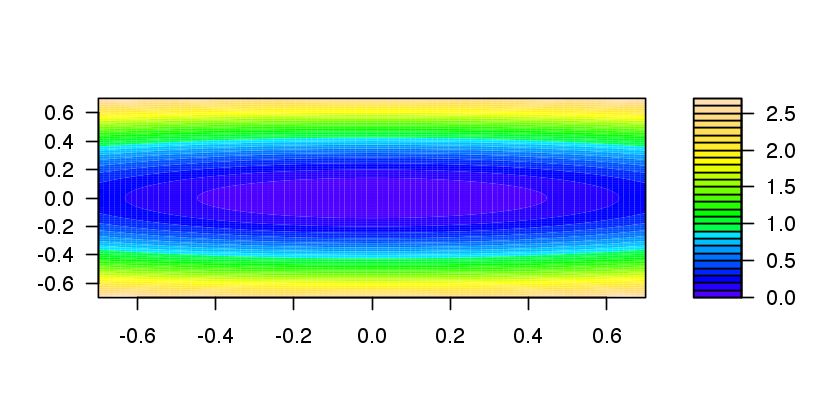In :
GradF = function(x) {c(x, eta*x)}


The step size should satisfy $\tau_k < 2/\eta$. We use here a constant step size.

In :
tau = 1.8/eta


Exercise 1

Perform the gradient descent using a fixed step size $\tau_k=\tau$. Display the decay of the energy $f(x^{(k)})$ through the iteration. Save the iterates so that |X(:,k)| corresponds to $x^{(k)}$.

In :
source("nt_solutions/optim_1_gradient_descent/exo1.R")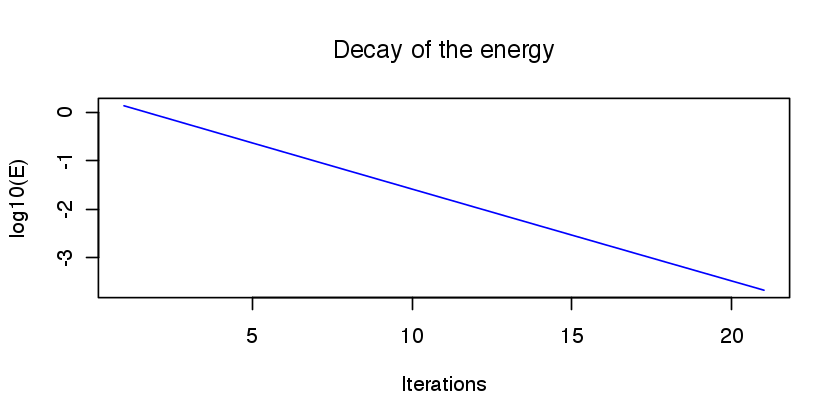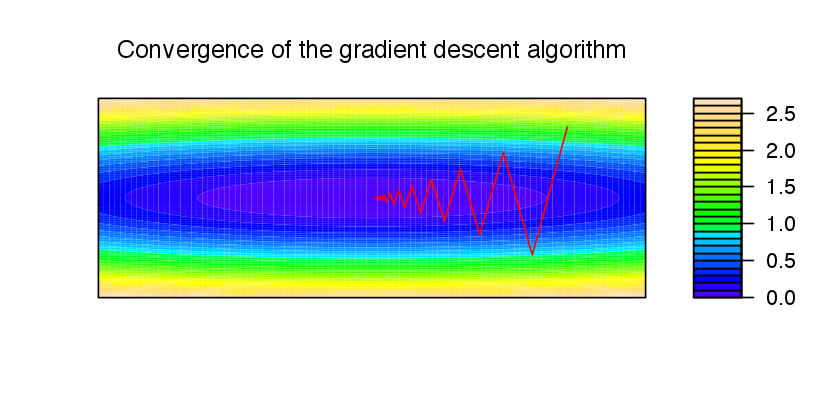In :
# Insert code here.


Display the iterations.

In :
# Insert code here.


Exercise 2

Display the iteration for several different step sizes.

In :
source("nt_solutions/optim_1_gradient_descent/exo2.R")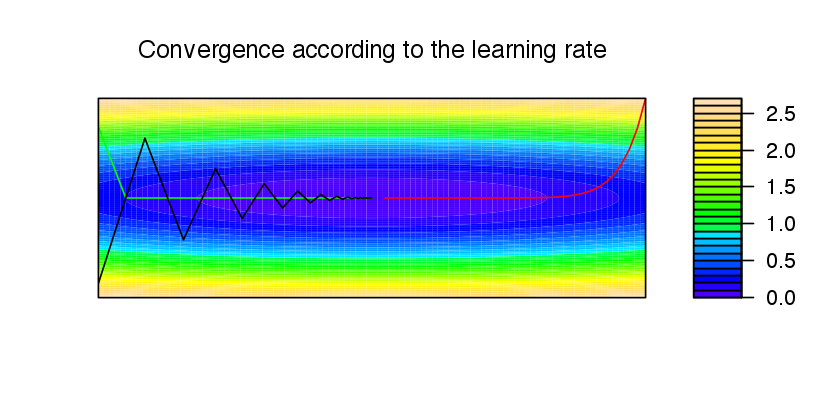In :
# Insert code here.


## Gradient and Divergence of Images¶

Local differential operators like gradient, divergence and laplacian are the building blocks for variational image processing.

Load an image $x_0 \in \RR^N$ of $N=n \times n$ pixels.

In :
n = 256
name = 'nt_toolbox/data/lena.png'


Display it.

In :
options(repr.plot.width=4, repr.plot.height=4)
imageplot(x0)For a continuous function $g$, the gradient reads $$\nabla g(s) = \pa{ \pd{g(s)}{s_1}, \pd{g(s)}{s_2} } \in \RR^2.$$ (note that here, the variable $d$ denotes the 2-D spacial position).

We discretize this differential operator on a discrete image $x \in \RR^N$ using first order finite differences. $$(\nabla x)_i = ( x_{i_1,i_2}-x_{i_1-1,i_2}, x_{i_1,i_2}-x_{i_1,i_2-1} ) \in \RR^2.$$ Note that for simplity we use periodic boundary conditions.

Compute its gradient, using finite differences.

In :
grad = function(x){
n = dim(x)
hdiff = x[,] - x[c(n, 1:n-1),]
vdiff = x[,] - x[,c(n, 1:n-1)]
return (array(c(hdiff, vdiff), dim=c(n, n, 2)))
}


One thus has $\nabla : \RR^N \mapsto \RR^{N \times 2}.$

In :
v = grad(x0)


One can display each of its components.

In :
options(repr.plot.width=6, repr.plot.height=6)
imageplot(v[,,1], 'd/dx', c(1,2,1))
imageplot(v[,,2], 'd/dy', c(1,2,2))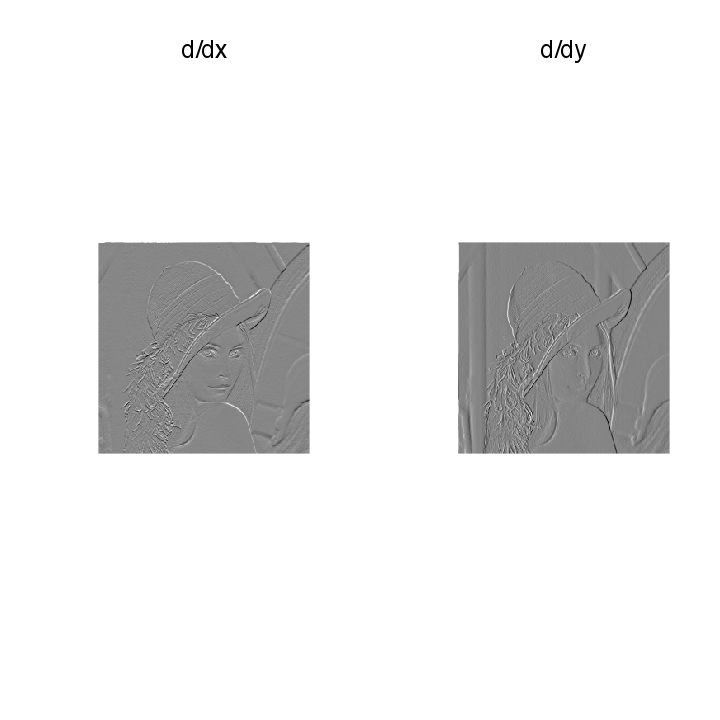One can display its magnitude $\norm{(\nabla x)_i}$, which is large near edges.

In :
options(repr.plot.width=4, repr.plot.height=4)
imageplot(sqrt((v * v)[, , 1] + (v * v)[, , 2]))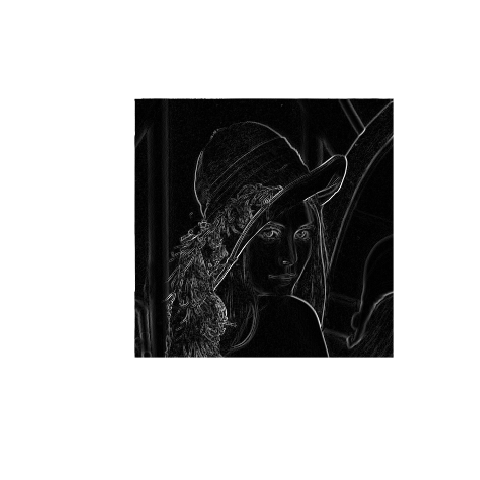The divergence operator maps vector field to images. For continuous vector fields $((v(s) \in \RR^2)$, it is defined as $$\text{div}(v)(s) = \pd{v_1(s)}{s_1} + \pd{v_2(s)}{s_2} \in \RR.$$ (note that here, the variable (s) denotes the 2-D spacial position). It is minus the adjoint of the gadient, i.e. $\text{div} = - \nabla^*$.

It is discretized, for $v=(v^1,v^2)$ as $$\text{div}(v)_i = v^1_{i_1+1,i_2} - v^1_{i_1,i_2} + v^2_{i_1,i_2+1} - v^2_{i_1,i_2} .$$

In :
div = function(x)
{
n = dim(x)
hdiff1 = x[c(2:n, 1), , 1]
hdiff2 = x[, c(2:n, 1), 2]
return(hdiff1 - x[,,1] + hdiff2 - x[,,2])
}


The Laplacian operatore is defined as $\Delta=\text{div} \circ \nabla = -\nabla^* \circ \nabla$. It is thus a negative symmetric operator.

In :
delta = function(x)
{

Display $\Delta x_0$.
imageplot(delta(x0))